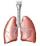# Prism - eq triangle

Calculate the volume and surface of the prism with the base of an equilateral triangle with side a = 4cm and the body height is 6cm.

Result

V =  41.569 cm3
S =  85.856 cm2

#### Solution:Leave us a comment of example and its solution (i.e. if it is still somewhat unclear...):

Showing 0 comments:Be the first to comment!#### To solve this example are needed these knowledge from mathematics:

Tip: Our volume units converter will help you with converion of volume units. See also our trigonometric triangle calculator.

## Next similar examples:

1. Triangular prismCalculate the volume and surface of the triangular prism ABCDEF with base of a isosceles triangle. Base's height is 16 cm, leg 10 cm, base height vc = 6 cm. The prism height is 9 cm.
2. Triangular prismCalculate the volume and surface area of a triangular prism if it is given: a = 6.8 dm. ..Va = 4 dm. (base edge length and base triangle height length) ... ... .v = 23 dm (body height)
3. Triangular prismCalculate the surface area and volume of a triangular prism, base right triangle if a = 3 cm, b = 4 cm, c = 5 cm and height of prism h=12 cm.
4. Triangular prismCalculate the volume of a triangular prism 10 cm high, the base of which is an equilateral triangle with dimensions a = 5 cm and height va = 4,3 cm
5. Triangular prismCalculate the surface of a regular triangular prism with a bottom edge 8 of a length of 5 meters and an appropriate height of 60 meters and prism height is 1 whole 4 meters.
6. Square prismCalculate the volume of a foursided prism 2 dm high, the base is a trapezoid with bases 12 cm, 6 cm, height of 4 cm and 5 cm long arms.
7. PoolThe prism-shaped pool is 2 m deep with a bottom of the isosceles trapezoid with base dimensions of 10 m and 18 m and arm legs 7 m long and 5.7 m long. During the spring cleaning, the bottom and walls of the pool must be painted. How many m2 of paint should
8. Regular triangular pyramidCalculate the volume and surface area of the regular triangular pyramid and the height of the pyramid is 12 centimeters, the bottom edge has 4 centimeters and the height of the side wall is 12 centimeters
9. TriangleCalculate heights of the triangle ABC if sides of the triangle are a=75, b=84 and c=33.
10. Parcelparcel has a rectangular shape of a trapezoid with bases 12 m and 10 m and a height 8 m. On parcel was built object with a footprint an isosceles triangle shape with side 4 m and height three-quarters of a meter. What is the area of unbuild parcel?
11. Center traverseIt is true that the middle traverse bisects the triangle?
12. Parallelogram ABCDThe area of parallelogram ABCD is 440 cm2. Points M and N are the midpoints of the sides AB and BC. What is the area of a quadrilateral MBND?
13. Cone area and sideCalculate the surface area and volume of a rotating cone with a height of 1.25 dm and 17,8dm side.
14. Cube volumeThe cube has a surface of 384 cm2. Calculate its volume.
15. Total displacementCalculate total displacement of the 4-cylinder engine with the diameter of the piston bore B = 6.6 cm and stroke S=2.4 cm of the piston. Help: the crankshaft makes one revolution while the piston moves from the top of the cylinder to the bottom and back.
16. Garden pondConcrete garden pond has bottom shape of a semicircle with a diameter 1.7 m and is 79 cm deep. Daddy wants make it surface. How many liters of water is in pond if watel level is 28 cm?
17. SportsmanA trained athlete is able to exhale after a deep breath still 500 ml of air. At normal inhalation and exhalation is breathing 500 ml of air. Within one minute, one breath and exhaled 14 times. What part of breathing air per day is one exhalation?